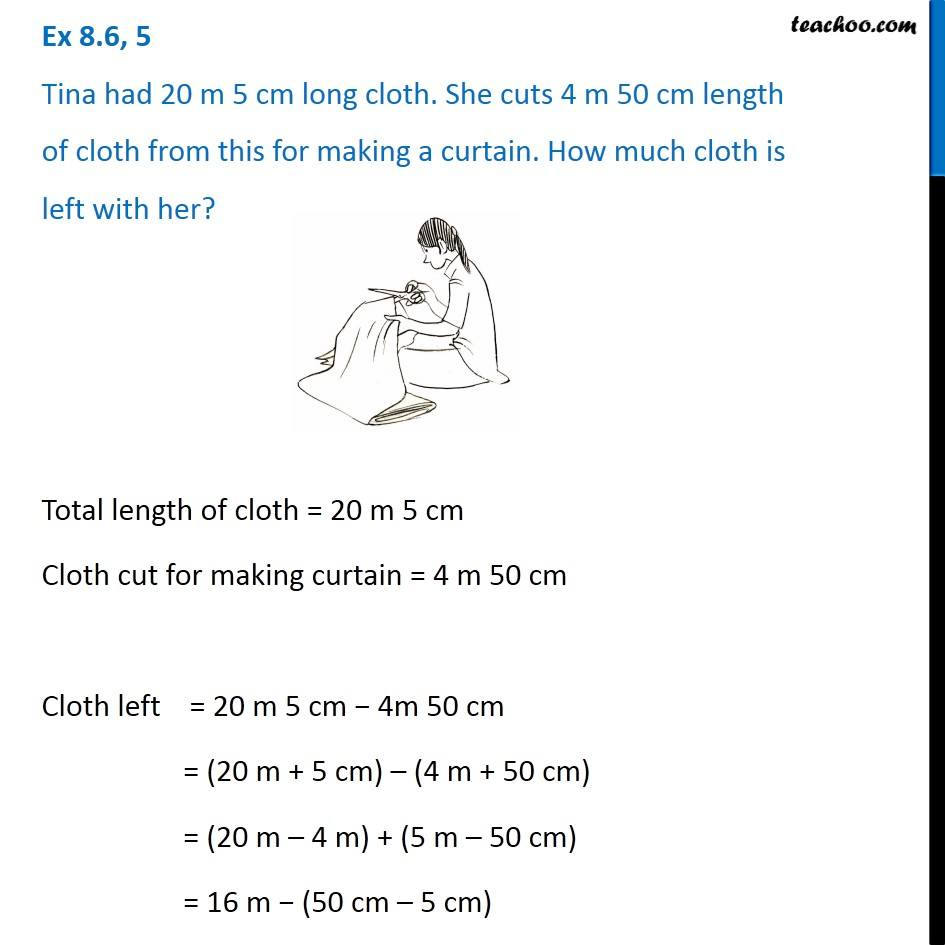Subtraction of decimal numbers - Statement questions

Chapter 8 Class 6 Decimals
Concept wiseLearn in your speed, with individual attention - Teachoo Maths 1-on-1 Class

### Transcript

Ex 8.4, 5 Tina had 20 m 5 cm long cloth. She cuts 4 m 50 cm length of cloth from this for making a curtain. How much cloth is left with her?Total length of cloth = 20 m 5 cm Cloth cut for making curtain = 4 m 50 cm Cloth left = 20 m 5 cm − 4m 50 cm = (20 m + 5 cm) – (4 m + 50 cm) = (20 m – 4 m) + (5 m – 50 cm) = 16 m − (50 cm – 5 cm) = 16 m − 45 cm = 16 m − 45 × 1 cm = 16 m − 45 × 1/100 m = 16 m − 45/100 m = 16 × 100/100 − 45/100 = 1600/100 − 45/100 = ((1600 − 45)/100) = 1555/100 = 15.55 m ∴ Cloth left = 15.55 m